International Journal of Materials Science and Applications
Volume 5, Issue 3, May 2016, Pages: 129-135

Study of Roll Forming Bending in Different Temperature

Ya Zhang, Dong-Won Jung*

Department of Mechanical Engineering, Jeju National University, Jeju-si, Republic of Korea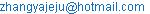(Ya Zhang)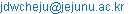(Dong-Won Jung)

*Corresponding author

Ya Zhang, Dong-Won Jung. Study of Roll Forming Bending in Different Temperature. International Journal of Materials Science and Applications. Vol. 5, No. 3, 2016, pp. 129-135. doi: 10.11648/j.ijmsa.20160503.13

Received: April 11, 2016; Accepted: May 20, 2016; Published: May 23, 2016

Abstract: Flexible roll forming is a high efficiency sheet metal forming process for producing variable cross section profiles. Also spring-back is one of the most important problems in flexible roll forming process. Spring-back is severe during the unloading phase of bending and greatly affects the forming accuracy of the bending parts. In this paper, the spring-back of the flexible roll forming in different temperature have been studied using finite element analysis. The simulation is elaborated by using ABAQUS software linked to elastic–plastic modules which we developed taking into account of interactions between these fields. According to finite element analysis results, the bending after roll forming has been analyzed. Therefore, we can make the comparison of the spring-back and forming force under different temperature from the finite element analysis. A flexible roll forming setup was developed and some parameters in the simulation have been discussed. AZ31 magnesium alloys were used in the simulation and assumed the material is isotropic.

Keywords: Flexible Roll Forming, Bending, Temperature, Finite Element

Contents

1. Introduction

Roll forming has been known as a high productive method for the production of profiles or welded tubes and pipes. However, since it is relatively poorly understood, an industrial practice has been largely restricted to empiricism and heuristic rules. To solve these problems and improve an effectiveness of the process design, a simulation program has been developed . The roll-bending process is an efficient technique for forming a metal plate into a cylindrical or conical hollow shape. It is widely used in today’s metal forming industry. There are many different types of roll benders available to plate bending manufacturers, including, for example, the three-roll pyramidal model, the three-roll pinching model, and the four-roll symmetric model .

The roll forming process is operated between roll forming multi-stages continuously. And a profile is formed in several forming steps from an unreformed strip to a finished profile without changing the thickness of the sheet. The scientific design of passes is carried out by combining the theoretical analysis with the finite element analysis in accordance with the principles of cold roll-formingthus the desirable high-quality bending sections achieved through the combination of the theory with practice.

Roll forming can also be implemented at a certain higher temperature to avoid the cracking of paints. With the thermal process, the sheet will become soft enough without crack, but hard enough not to be stripped off the metal. Also, in this way, the forming force of the metal will decrease especially for high tensile steel forming .

Wen Kang and Yixi Zhao  established an elastic-plastic roll forming finite element model with ABAQUS to investigate the influence of different material parameters. K. Richter and R. Haase  had presented a roll forming process with magnesium alloys can validated on a near-industrial scale with sheet metal strips and concluded out the temperature is one of the most significant impacts on process ability. Zhou Sui and Zhongyi Cai  had designed a continuous flexible roll bending process. The algorithms of the mathematical models were verified by comparisons of the desired and the numerical simulation results. Compare the experiment with the target shape, it shows that the software system is reliable.

Nowadays there are more and more demands on such sheet metal, especially for the ones produced in single-piece or small-batch quantity, however, the widely used sheet metal forming processes such as stamping, stretching and hydro-forming, etc. are only profitable for mass production, the large initial investments and long setup time make them unsuitable for manufacturing small-batch products, and the sheet metal forming process based on the principle of continuous forming and the usage of flexible forming tools will be an effective way to manufacture swept surface parts in the small-batch production.

Discontinuous manufacturing technologies, such as stamping and stretch-forming, produce a product at one time. The discontinuous flexible forming process, which is based on the usage of a reconfigurable die, does not require expensive conventional die and time-consuming setup operations, and thus is suitable and efficient for the small quantity production of doubly curved sheet metals.

Accurate production of designed parts is a main goal of the sheet metal industry, reducing the need for post forming processing resulting in cost and time reduction. The problems of post-forming operations are more pronounced for thick sheets due to the weight of bent parts. Springback is the recovery of the elastic part of the deformation after removal of the external load which is applied during the bending operation; this phenomenon is a major source of inaccuracies in a bending process. This indicates the complex nature of springback, depending on numerous material and process parameters. Currently, the accuracy of spring back simulation has become an important symbol of finite element simulation software technology level in sheet forming process. Spring-back will increase the cost of resilience test, repair and calibration mode shape workload; it is forced in production cut this need to take effective measures. However, the conventional analytical method and empirical formula cannot accurately calculate the amount of the spring-back plate material after unloading. At present, computer simulation technology is recognized as a new and reliable method for solving the spring-back problem.

In this study, flexible roll forming bending process is designed to predict the bending process and the sheet mechanical behavior in different temperature. The stress distribution and energy change have been analyzed.

2. Objectives and Approach

The main objective of this study is to develop of a flexible roll forming bending process for an irregular sheet to achieve a constant bending width. Also the mechanical behavior and spring-back have been investigated, as a case study for the flexible roll forming process.

The CAD model of the desired shape was generated by the Solidworks and the simulation was carried out using a commercial finite simulation program ABAQUS.

The steps of this investigation were:

1. Model a given sheet which has a constant bending width and determines the rolls shape and dimension.

2. Define the roll forming process and conditions in ABAQUS such as mesh and interactions.

3. Determine the rotation center of the rolls and establish the boundary conditions for the rolls to make them moving forward as desired.

4. Evaluate effects of the process parameters based on the simulation results and determine the optimal positions and boundary conditions of the rolls.

3. Finite Element Model

The channel material is common structural sheet magnesium AZ31 with a Young’s modulus of 44.8 (GPa) and Poisson’s ratio of 0.35 . The simulation parameters used in finite element model are given in table 1 and the true stress-strain curves under different temperatures are displayed in Figure 1. The yield stress decreases with the increase of the temperature. Usually, with the increase of temperature, the recovery of metal crystals happened and recrystallization can decrease the hardening of the strain. Also, the movement of crystals become more frequent and results in the decrease of critical shear stress. The material used in this simulation is considered to be isotropic on both elastic property and plastic property.

Table 1. Simulation parameters used in finite element model.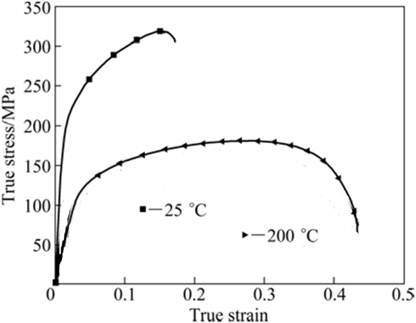Figure 1. True plastic strain (mm/mm).

With the different design of rolls, the sheet could be deformed successively into products with a different section. Three experimental setups of flexible roll forming process are modeled. For all sheets have a width of 900mm and the length in the longitudinal direction is 107.5mm. For this particular task a symmetrical model could be implemented. The deformed sheet progressively passes through a series of rolls. The angles of the rolls are from left to right, 15°, 30° and 45°. The metal sheets with the thickness of 1mm are used as the initial metal strip. The parameters of the sheet are presented in Figure 2. The thickness of the sheet is 1mm. There is only half of the sheet shown in the figure and the sheet is a rectangle with a 3-degree edge in both two sides.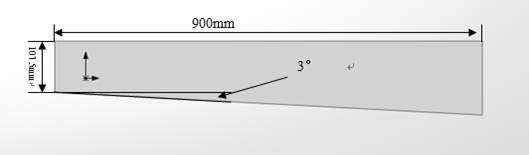Figure 2. Dimension of sheet with 3 degree.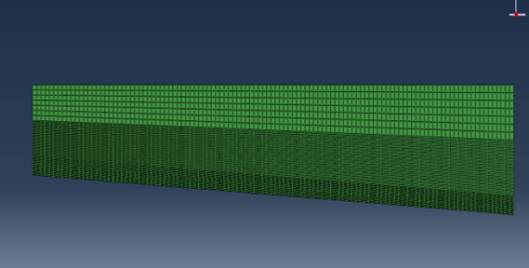Figure 3. Mesh of sheet with 3 degree.

4. Design of Roll Forming Process

The continuous flexible roll bending process is complicated from a finite element (FE) perspective. Its features include contact phenomena, large strain plasticity, and large displacements. Hence, the following assumptions were applied for the purpose of computational efficiency :

1. The flexible rolls were conceived as rigid bodies.

2. The material of the sheet metal was assumed to be homogeneous, with a bilinear stress-strain relationship.

3. The weight of the sheet metal was neglected.

4. The static and dynamic friction coefficients were considered to be constant on the contact lines between the flexible rolls and the sheet metal.

5. Plane strain conditions prevailed.

Element types, the number of elements, and mesh quality are also important in forming process. For the shell model, S4R elements were used: a 4-node doubly curved thin or thick shell, reduced integration, hourglass control and finite membrane strains. An important factor for the springback calculation is the number of integration points through the thickness of an element. However, the calculation time for the shell model through the thickness would make a huge increase in calculation time. In this simulation, there would be 3 integration points across the thickness direction. The sheet has been divided into two parts for the mesh. After meshing, the 900 mm long half shape sheet contained 5276 elements. The mesh of the sheet is shown in Figure 3. The sheet in this simulation is symmetry, so only half of the sheet is used. The bending zone has more elements than the web zone and flange zone. In this way, the result will be accurate enough and also same simulation time. Also, the mass scaling is used in this simulation to reduce the simulation time and the scaling factor is 100. For all models, the solution has been implemented in two steps: an explicit bending step followed by an implicit springback step. Afterwards, the results from an output database have been processed and the bend angles as well as the springback angle were determined .

In the sheet forming process, the Explicit, Dynamic step will be used. The step time is 1 second. For the explicit integrate calculation, a certain material property such as density, Young’s modulus and Poisson’s ratio, the minimum step time Δtmin is depended on the minimum element length lmin and velocity of sound C.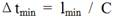(1)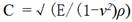(2)

From the Equation 1 and Equation 2, we can know that for a designed mesh, the minimum step time Δtmin is depended on the velocity of sound C. When the step time becomes very small, the total time for the simulation will be much longer. So the mass scaling will be used to increase the physical density to increase the step time. In this way, we can speed up the computation. So, there has a mass scaling factor of 100 is used in the simulation to accelerate the computation time with decrease the computation precious.

There are three possible ways to control the rigid contact bodies, by referencing the velocity, rotation and displacement or load. Each way can be chosen to execute the movement of the flattener rolls. For the sheet which has an angle of 3 degrees is easier to use the velocity method by defining the velocity in X-axis and in addition with Y-axis.

The control of positioning of the sheet is conducted by defining a rotation in the Y direction. The movement in X and Y direction are fixed. The boundary conditions are performed by defining the centerline of the sheet. After defining these nodes for the sheet, the necessary movement definitions were given in the boundary condition tables. The boundary conditions are applied in the reference point of the rigid body. The position of the rolls is given in Figure 4. The distance between the roll is constant for 200mm.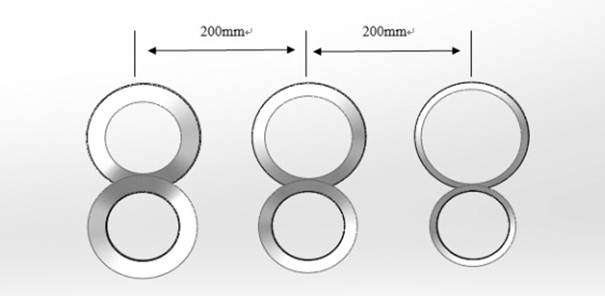Figure 4. Distances between rolls.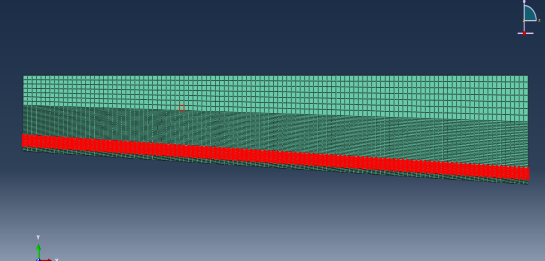Figure 5. Heating region of the sheet.

After introducing the boundary conditions, contacts were defined to present the contacts between the rigid bodies and deform bodies. The interactions between them were surface to surface contact and definite by the step. It has been assumed that the friction between the roll and sheet is penalty and the friction coefficient is zero. According to the research of the Q. V. Bui and J. P. Ponthot , the frictions in the simulation have no significant influence on the simulation result such as spring back and bowing. In the other side, the existence of friction in the simulation may affect the poison accuracy of the rolls and sheet.

The objectives studied in this paper can be classified into two general categories. The first category includes parameters such as material property, blank thickness that are shared between this method and conventional processes. In the second category, to study the effects of spring-back parameters, the change of temperature is taken into consideration for bending part as depicted in Figure 5. We assumed that there are no other kinds of heat transfer in the simulation and the temperature in the region with red color is 250 degree. And this region is also the region where bending happened. Other parameters are as same with the cold roll forming.

5. Process and Simulation

The geometry of the strips after rolling deformation is shown in Figure 6 and Figure 7. The forming result is influenced by the thickness, the material property, the roll diameter, the deformation rate of the strip, and the angular velocity of the roll. In the simulation, only deformation areas are considered. The tensions before and behind of the deformation area are not considered.

The distributions of residual stress and equivalent plastic strain are shown in Figure 6 and Figure 7, which reveal that the maximum stress and strain are located in the bending region. The stress mainly on the flange zone and the web zone have a much more stress because in this simulation, the friction is considered to be zero and there is no thickness change in the web zone.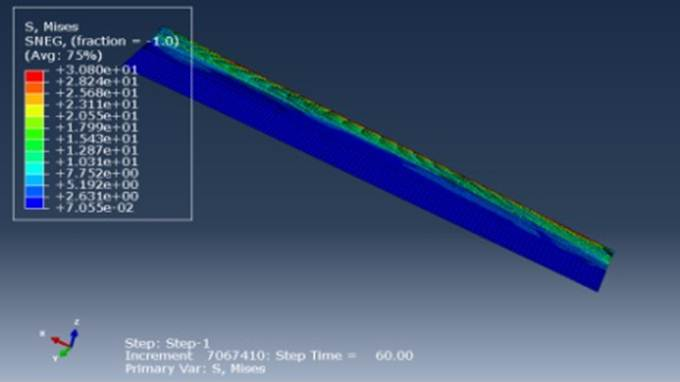Figure 6. Strain distribution after roll forming with heating.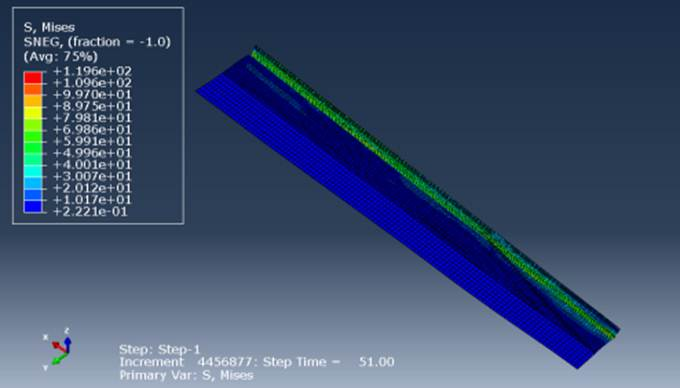Figure 7. Strain distribution after roll forming without heating.

To analyze the effect of the flexible roll forming, the logarithmic strain during roll forming is investigated. The comparison of the logarithmic strain of the element is shown in Figure 8 and Figure 9. As the initial metal strip is passing through the roll stands, the increase of logarithmic strain distribution of the roll-formed profile is accumulated by the incremental increase of the logarithmic strain at each forming roll as shown in Figure 8 and Figure 9. The logarithmic strain at each forming roll increases. In our study, the peak logarithmic strain occurs just ahead of each roll station during flexible roll forming. However, the increase of the logarithmic strain in the sheet without heating is 10-11 times larger than the logarithmic strain in the sheet with heating.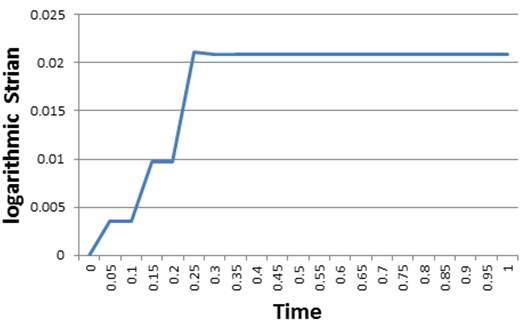Figure 8. Logarithmic strain after roll forming with heating.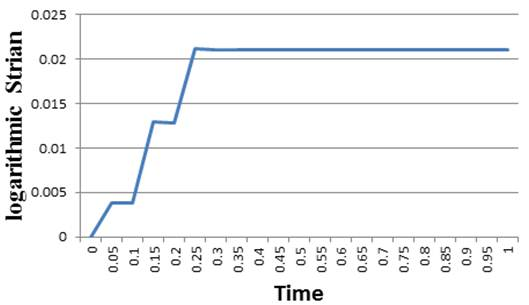Figure 9. Logarithmic strain after roll forming without heating.

Figure 10 and Figure 11 shows the PEEQ (equivalent plastic strain) of the same element at the end of the sheet within the bending zone. The PEEQ stands for the plastic strain in the forming process. The strain in the sheet with heating increase earlier than the one without heating But they come to a same level of strain in the end. With the roll forming process, the strain increase. We can know from the figure that the thermal process has no influence on the strain change.Figure 10. PEEQ after roll forming with heating.Figure 11. PEEQ after roll forming without heating.

Energy calculation is one of the most important parameters in the simulation process. To analyze the different kinds of energy in the forming process can help evaluate the reliability of the simulation process. For the whole model, the energy balance equation can be written as following:

EI + EV + EFD + EKE – EW = E total = constant      (3)

In this equation, EI stands for the internal energy, EV is kinetic energy, EFD is frictional dissipation, EKE is viscous dissipation and EW is external work. The total of this energy must be a constant. In this paper, we are mainly focused on the internal energy and kinetic energy. For the EW, it can be considered as the energy produced by the rolls.

As illuminated in Figure 12 and Figure 14, the internal energy is increased with the time. With the deformation happened, the internal energy becomes bigger because of the external work of the rolls. The internal energy with high temperature is higher than the sheet in the room temperature. This is because of the high temperature increase the internal energy by increase the crystals movement. Figure 13 and Figure 15 shows the kinetic energy of the two forming models. The kinetic energy both in the room temperature and high temperature are stable around the simulation. We can calculate out that in both models, the kinetic energy is less than 5% of the internal energy. Which means the simulation is under a considerable condition. The mass scaling used in the simulation is acceptable under this condition. Although the kinetic energy is not so stable around the roll forming process, the result is also reliable.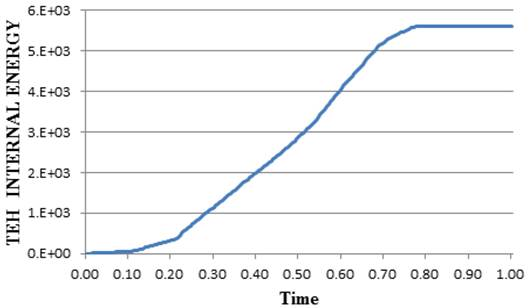Figure 12. Internal energy without heating.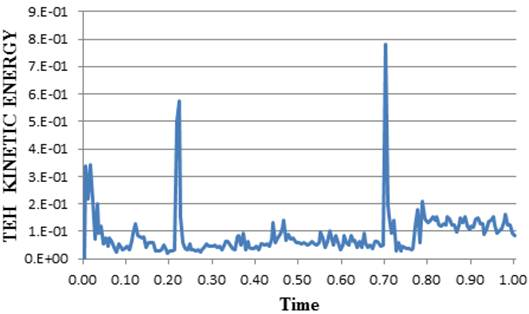Figure 13. Kinetic energy without heating.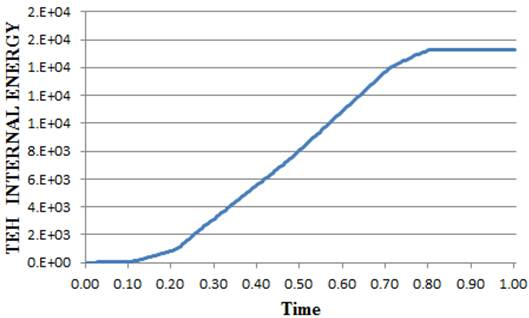Figure 14. Internal energy with heating.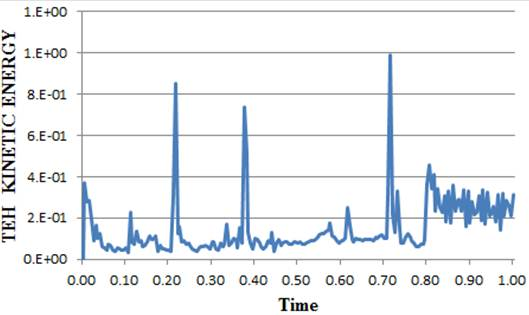Figure 15. Kinetic energy with heating.

Applying a suitable elastic–plastic deformation is essential to gain the desired geometry in sheet metal forming processes. After completion of the process and during elimination of forming loads, the elastic portion of total imposed strain is recovered and leads to a dimensional deviation in formed part. This phenomenon is known as spring-back that can directly influence the dimensional accuracy and geometry of desired workpiece which leads to major incompatibilities in assembly phase . Therefore, the quantitative make a prediction precisely and compensation of spring-back is a crucial stage in die designing process.

As shown in Figure 16, the elastic recovery happened after bending process, the s is sheet thickness and the α1 stands for the required bending angle, α2 is the desired angle. In the metal forming process, the spring-back is very important for the forming process and has a deep influence on the forming precious. The shape of the part is decided by the spring-back angle, when the angle is beyond the tolerance, defect happened. Therefore, the spring-back has been an important factor restricting the die and product quality. With the development of the automotive and aerospace industry in recent years, especially due to the widely use of the high strength steel and aluminum sheet . The problem of spring-back becomes more and more important.

The simulation uses the Static, General step to calculate the springback angle. All rigid bodies have been removed. Only the symmetry boundary condition left. The result of the roll forming has been imported into the simulation as an initial state. The step time for the simulation is 1second.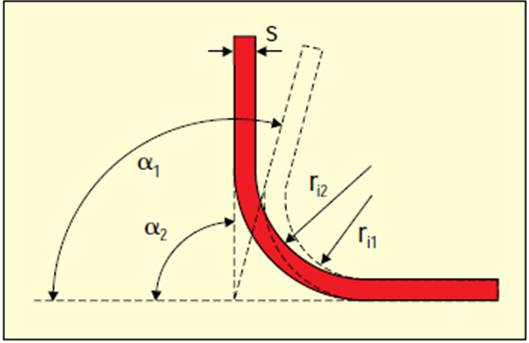Figure 16. The spring back of the sheet.

The simulation gets seven points along the sheet side to get the amount of spring-back angle. The spring-back points and angle are shown in Figure 17 and Figure 18. This phenomenon can be explained that by increasing the temperature of the sheet, the required force for forming process is decrease due to the smaller resistance of harder forming pressures. As it can be inferred, by using a sheet with higher temperature the workpiece experiences a greater thickness strain in bending radius area that decreases the ratio of elastic deformation to the total amount of deformation and consequently the reduction of spring-back.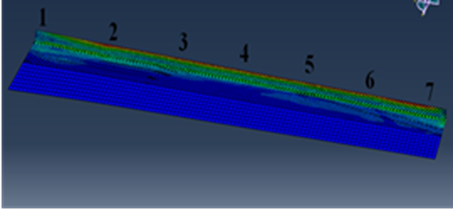Figure 17. Measurement points of the spring-back angle.Figure 18. The comparison of the spring-back angle.

Spring-back is affected by many factors. Temperature is the only one of the parameters which affects the spring-back angle. The yield strength (δs), the ratio between the radius of the die and thickness and the bending angle also has a certain influence on the spring-back angle.

6. Conclusion

In this paper, two flexible roll forming bending processes of AZ31 magnesium alloy with different temperature of the sheet were analyzed and we can conclude that using this model can effectively predict the trends in the distribution of the logarithmic strain and spring-back. The internal energy and kinetic energy also have been analyzed. Through the simulation program, important parameters are determined by taking account of roll stand distance and roll forming speed. With the study above, we can know that:

1. Through the rigid-plastic finite element method, the flexible roll forming process can be effectively analyzed. The forming process can be effective learned.

2. The logarithmic strain has been learned in the paper and both in the sheet with heating and without heating, the logarithmic increase with the stages and all of them are under the certain limit.

3. The roll forming with higher temperature has a smaller spring–back angle compares to the one which in room temperature.

4. The above simulation results can be utilized in the real roll forming industry. It is either done by choosing suitable metal strips, or by modifying the process parameters, such as the roll design.

Spring-back is a complex problem and depends on many factors. This paper just analyzes some factors and the influence of them. Other parameters such as material property, blank thickness, friction type and anisotropy also have a significant influence on the spring-back in flexible roll forming. If take consider of these parameters, we will have a more accurate result.

Acknowledgements

This research was supported by Basic Science Research Program through the National Research Foundation of Korea (NRF) funded by the Ministry of Education (2014009199).

References

1. Sukmoo Hong, A parametric study on forming length in the roll forming. Journal of Material Processing Technology. Volume 113, pp. 774~778. (2001).
2. Jun Zeng, FEM dynamic simulation and analysis of the roll-bending process for forming a conical tube. Journal of Material Process Technology.198 (2008) , pp. 330-343.
3. Xuechun Li, Effect of the material-hardening mode on the springback simulation accuracy of V-free bending. Journal of Material Processing Technology. 123(2002) , pp. 209 -211.
4. Wen Kang, Yixi Zhao, Numerical simulation and parameters analysis for roll forming of martensitic steel MS980. Procedia Engineering 81(2014), pp. 251-526.
5. K. Richter and R. Haase, Tempered forming of magnesium alloy using the example of roll forming. Materials Today: Proceedings, 2s (2015), pp. 60-66.
6. Zhou Sui, Zhongyi Cai, Simulation and software design of continuous flexible roll bending process for three dimensional surface parts. Materials and Design 54 (2014) pp. 498-506.
7. Xiao Han, Springback characteristics of AZ31 magnesium alloy as-extrude profile in warm tension-rotation bending process. Trans actions of Nonferrous Me tals Soc iety of China 22 (2012), pp. 416- 421.
8. Zhou Sui, Simulation and software design of continuous flexible roll bending process for three dimensional surface parts. Materials and Design. 54 (2014) , pp. 498-50 0.
9. Vitalii Vorkov, Springback prediction of high-strength steels in large radius air bending using finite element modelling approach. Procedia Engineering. 81 (2014) , pp. 1005–1010.
10. Q. V. Bui, J. P. Ponthot, Numeriacal simulation of cold roll forming process. Journal of materials processing technology 202 (2008) , pp. 2785-282.
11. Mahmoud Yaakobi, Simulation of the thermo mechanical and metallurgical behavior of steel by using ABAQUS software. Computational Materials Science. 68 (2013), pp. 297-306.
12. Q. V. Bui, Numerical simulation of cold roll-forming processes. Journal of materials processing technology 20 (2008), pp. 275-285.

 Contents 1. 2. 3. 4. 5. 6.
Article ToolsAbstractPDF(1941K)